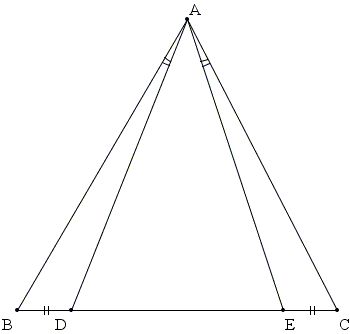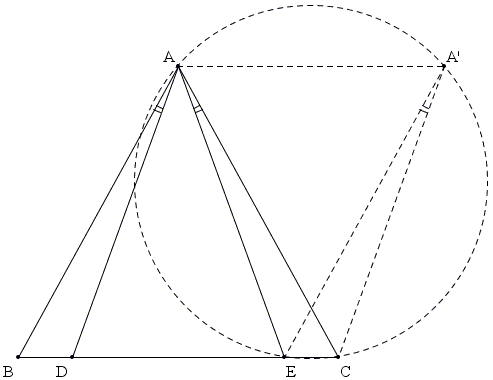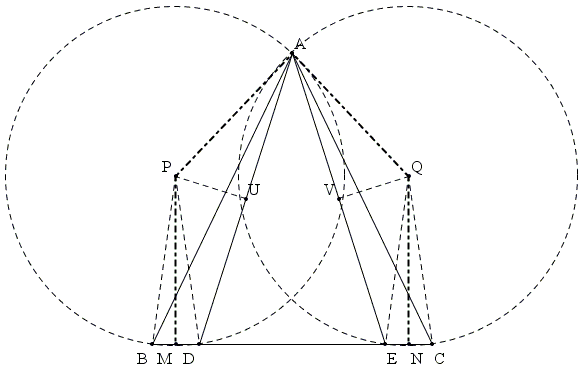# Phantom Circle and Recaptured Symmetry

In ΔABC, D and E are two points in the interior of side BC such that BD = CE and ∠BAD = ∠CAE. Prove that ΔABC is isosceles.SolutionCopyright © 1996-2018 Alexander Bogomolny

In ΔABC, D and E are two points in the interior of side BC such that BD = CE and ∠BAD = ∠CAE. Prove that ΔABC is isosceles.This problem has been offered at the 2007 Bay Area Mathematical Olympiad. To quote from [Stankova and Rike, p. 225], Of the 157 solutions, about one third received perfect or near perfect scores. Yet, just about every solution used ideas entrenching its author in unsightly calculations with trigonometry or analytic geometry. Very few (if any) students saw glimpses of the truly beautiful and elegant geometry of the problem. What was so hard to see here?

### Solution 1The authors observe that the condition of a segment subtending an angle is begging for a circle to which both are inscribed. To obtain such a circle they translate ΔABD into ΔA'EC. Now four points A, E, C, A' are concyclic while AA'||EC, making quadrilateral AECA' an isosceles trapezoid. The presence of the phantom circle immediately resolves the problem: AC = EA' = AB, proving that ΔABC is isosceles.

### Solution 2

Another solution appeals to the fact that a line segment and an angle define a circle (or rather two circular arcs - but this is not important here). The meaning of this statement is that the two given quantities (the length of the segment and the measure of the angle) define uniquely the radius of a circle and its center.It then follows that the circumcircles of triangles ABD and AEC are equal. If M and N are the midpoints of BD and EC then the pentagon MPAQN is symmetric with respect to the perpendicular from A to BC. (This is because, MPQN is a rectangle, while ΔMAN is isosceles.)

The symmetry implies the equality of all related segments, AB and AC, in particular.

### References

1. A Decade of the Berkeley Mathematical Circle, The American Experience, Volume I, Z. Stankova, Tom Rike (eds), AMS/MSRI, 2008### Chasing Inscribed AnglesCopyright © 1996-2018 Alexander Bogomolny

 65270779